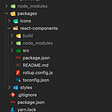# Instancing with three.js — Part 2

`console.log(renderer.info.render.calls) //add right above the render callrenderer.render( scene, camera );`
`var geometry = new THREE.BoxBufferGeometry( 20, 20, 20 );for ( var i = 0; i < 2000; i ++ ) {var object = new THREE.Mesh( geometry, new THREE.MeshLambertMaterial( { color: Math.random() * 0xffffff } ) );object.position.x = Math.random() * 800 - 400;     object.position.y = Math.random() * 800 - 400;     object.position.z = Math.random() * 800 - 400;object.rotation.x = Math.random() * 2 * Math.PI;     object.rotation.y = Math.random() * 2 * Math.PI;     object.rotation.z = Math.random() * 2 * Math.PI;object.scale.x = Math.random() + 0.5;     object.scale.y = Math.random() + 0.5;     object.scale.z = Math.random() + 0.5;scene.add( object );}`
`var geometry = new THREE.BoxBufferGeometry( 20, 20, 20 );var instancedGeometry = new THREE.InstancedBufferGeometry() //this is going to wrap both geometry and a bit of the scene graph//we have to copy the meat - geometry into this wrapperObject.keys(geometry.attributes).forEach(attributeName=>{  instancedGeometry.attributes[attributeName] = geometry.attributes[attributeName]})//along with the indexinstancedGeometry.index = geometry.index`
`instancedGeometry.maxInstancedCount = 2000`
`const matArraySize = 2000 * 4const matrixArray = [  new Float32Array(matArraySize),  new Float32Array(matArraySize),  new Float32Array(matArraySize),  new Float32Array(matArraySize),]`
`for( let i = 0 ; i < matrixArray.length ; i ++ ){   instancedGeometry.addAttribute(       `aInstanceMatrix\${i}`,       new THREE.InstancedBufferAttribute( matrixArray[i], 4 )    )}`
`const instanceColorArray = new Uint8Array(2000*3)instancedGeometry.addAttribute(  'aInstanceColor',  new THREE.InstancedBufferAttribute( instanceColorArray, 3, true ))`
`for ( var i = 0; i < 2000; i ++ ) {   var object = new THREE.Object3D() //we'll swap out the mesh with this//we should keep the color though  const color = new THREE.Color(Math.random() * 0xffffff)//we're going to piggy back of the scene graph and keep this as is  object.position.x = Math.random() * 800 - 400;  object.position.y = Math.random() * 800 - 400;  object.position.z = Math.random() * 800 - 400;object.rotation.x = Math.random() * 2 * Math.PI;  object.rotation.y = Math.random() * 2 * Math.PI;  object.rotation.z = Math.random() * 2 * Math.PI;object.scale.x = Math.random() + 0.5;  object.scale.y = Math.random() + 0.5;  object.scale.z = Math.random() + 0.5;scene.add( object );object.updateMatrixWorld() //we compute the matrix based on position, rotation and scale//now that we have the matrix computed we need to transfer it to the attribute    for ( let r = 0 ; r < 4 ; r ++ )  for ( let c = 0 ; c < 4 ; c ++ ){    matrixArray[r][i*4 + c] = object.matrixWorld.elements[r*4 + c]  }//same goes for color  const colorArray = color.toArray().map(c=>Math.floor(c*255))for( let c = 0 ; c < 3 ; c ++ )    instanceColorArray[i*3+c] = colorArray[c]}`
`instanceMaterial.onBeforeCompile = shader=>{shader.vertexShader = `attribute vec4 aInstanceMatrix0;attribute vec4 aInstanceMatrix1;attribute vec4 aInstanceMatrix2;attribute vec4 aInstanceMatrix3;attribute vec3 aInstanceColor;\${shader.vertexShader.replace(  '#include <begin_vertex>',  `     mat4 aInstanceMatrix = mat4(        aInstanceMatrix0,        aInstanceMatrix1,        aInstanceMatrix2,        aInstanceMatrix3     );vec3 transformed = (aInstanceMatrix * vec4( position , 1. )).xyz;  `)}}`
`shader.vertexShader = `varying vec3 vInstanceColor;\${  shader.vertexShader.replace(  `#include <color_vertex>`,  `#include <color_vertex>   vInstanceColor = aInstanceColor;  `)}``
`shader.fragmentShader = `     varying vec3 vInstanceColor;     \${       shader.fragmentShader.replace(       'vec4 diffuseColor = vec4( diffuse, opacity );',       'vec4 diffuseColor = vec4( vInstanceColor, opacity );'     )}``
`shader.vertexShader = shader.vertexShader.replace(       `#include <beginnormal_vertex>`,       `       mat4 _aInstanceMatrix = mat4(      aInstanceMatrix0,      aInstanceMatrix1,      aInstanceMatrix2,      aInstanceMatrix3       );       vec3 objectNormal = (_aInstanceMatrix * vec4( normal, 0. ) ).xyz;       `)`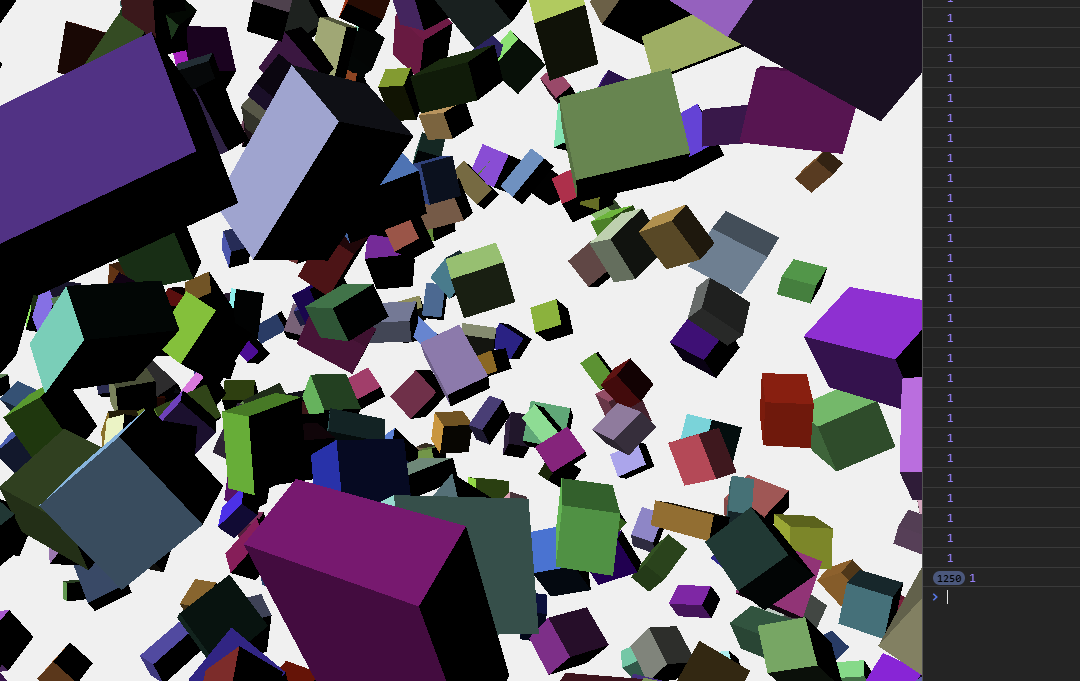Still at one draw call, the lighting is a bit more consistent

# CPU side stuff

`var camera, scene, raycaster, renderer;var intersectsScene`
`intersectsScene = new THREE.Scene()for ( var i = 0; i < 2000; i ++ ) {`
`const object = new THREE.Mesh(geometry)object.userData.index = iintersectsScene.add( object )`
`intersectsScene.updateMatrixWorld(true)`
`var intersects = raycaster.intersectObjects( intersectsScene.children );`
`// if ( INTERSECTED ) INTERSECTED.material.emissive.setHex( INTERSECTED.currentHex );`
`var container, stats;var camera, scene, raycaster, renderer;var intersectsSceneconst red = [255,0,0] //a constant colorconst instanceEmissiveArray = new Uint8Array(2000*3) //the emissive arrayconst emissiveAttribute = new THREE.InstancedBufferAttribute( instanceEmissiveArray, 3, true )emissiveAttribute.dynamic = true`
`function setEmissiveAtIndex( index, colorArray ) {    for( let i = 0 ; i < 3 ; i ++ ){     instanceEmissiveArray[index*3 + i] = colorArray[i]    }}function getEmissiveAtIndex( index ) {    const res = []    for( let i = 0 ; i < 3 ; i ++ ){     res.push(instanceEmissiveArray[index*3 + i])    }    return res}`
`instancedGeometry.addAttribute(   'aInstanceEmissive',   emissiveAttribute)`
`shader.fragmentShader = `     varying vec3 vInstanceEmissive;     \${       shader.fragmentShader.replace(       'vec3 totalEmissiveRadiance = emissive;',       'vec3 totalEmissiveRadiance = vInstanceEmissive;'     )}     ``
`// if ( INTERSECTED ) INTERSECTED.material.emissive.setHex( INTERSECTED.currentHex );if ( INTERSECTED ) setEmissiveAtIndex(  INTERSECTED.userData.index,   INTERSECTED.currentColorArray )`
`INTERSECTED.currentColorArray = getEmissiveAtIndex(INTERSECTED.userData.index)`

--

--

--

## More from Dusan Bosnjak

i like computer graphics

Love podcasts or audiobooks? Learn on the go with our new app.

## Breaking Changes to the MetaMask Inpage Provider## Angular Unit Test Automation## Getting started with Reanimated 2 for React native Part-1## Test Driven Development with Alexa SDK## 5 Free Ways to Get into Web Development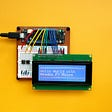## BRT.CSS Slideshow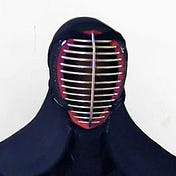## Dusan Bosnjak

i like computer graphics

## 🗿📃✂️🖖🦎”Rock Paper Scissors Spock Lizard” RPSSL Game 🕹️## Build Blog Site using Gatsby JS — Part 3 (Gatsby Plugin Mdx)## Yarn, npm, or pnpm?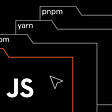## How to Create a React Multi-package UI Library